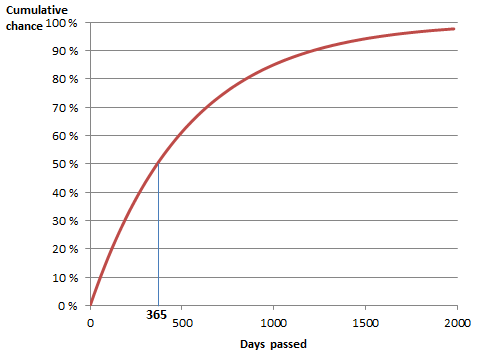欢迎访问欧陆风云4百科！注册一个账号，一起参与编写吧！这里是当前的工程。 2019年8月服务器费用已由站长支付，若您想帮助我们可以点这里！欢迎所有对百科感兴趣的同学加入QQ群：602439518。

# 事件

## 周期性事件

The events are grouped together into different sets depending on their purpose (regular gameplay or mechanic related) and assigned weights within their respective sets. Each set is programmed to fire in regular time intervals. The time intervals range from yearly intervals and up-to 5 year intervals. It is important to note however that there are several same-year intervals and it does not mean they'll fire at the same time; for example, there can be two 5 year intervals firing in consecutive years. When the interval arrives an event is picked at random from within the set and fired. Events with higher weights have higher chances to fire more commonly than the lower ones. Having certain modifiers, idea groups, provinces etc. can increase or decrease some events' weights.

## 基于MTTH（平均发生时间）的事件The chance of an event with an MTTH of 365 days firing within x days.

Other events are not triggered but have a chance of happening at any time if their conditions are met. These events are controlled by a mean time to happen (MTTH).

This mechanic determines the time it takes for an event to trigger, measured in months. Yet since events are probabilistic, there is a small chance an event may happen very quickly, or possibly never at all during the game. For example, if an event had a MTTH of 120 months, it means that usually it would take around a decade for the event to trigger. Certain factors may increase or decrease MTTH for an event, such as country's leader's quality, national stability, and so on.

Assuming the probabilities are calculated in the same manner as in EU3, then despite the name MTTH is actually the median time, or half-life, for the event to trigger, i.e., the amount of time during which there is a 50% chance of the event occurring by that time, rather than the mean (average).

The engine checks whether an event occurs every EVENT_PROCESS_OFFSET days, set to 20 at default. The chance per check is

$1-2^{-t_{c}/t_{1/2}}$where $t_{c}$is the EVENT_PROCESS_OFFSET and $t_{1/2}$is the median.

This approximates the "ideal" probability

$1-2^{-t/t_{1/2}}$where $t$is the amount of time that passes. The approximation is best for a long MTTH.

The median $t_{1/2}$and mean ${\overline {t}}$are related by

${\overline {t}}={\frac {t_{1/2}}{\ln 2}}\approx 1.44t_{1/2}$In terms of the mean, the ideal probability is:

$1-e^{-t/{\overline {t}}}$For a repeating event the number of occurrences within a given number of checks is given by a binomial distribution, which may be approximated by a Poisson distribution for a long MTTH.

## 另见

 君主能力 君主 • 顾问 • 理念组 • 国家理念 • 科技 • 稳定度 • 政策
 其它 决议 • 任务 • 事件 • 修正 • 灾难 • 政府 • 派系 • 威望 • 思潮 • 过度扩张 • 厌战度 • 力量投射
1. For a list of Pulse events see /Europa Universalis IV/common/on_actions/00_on_actions.txt.